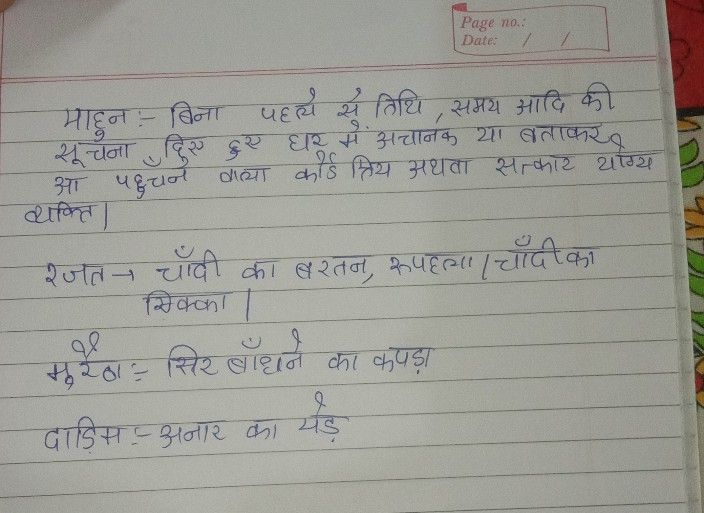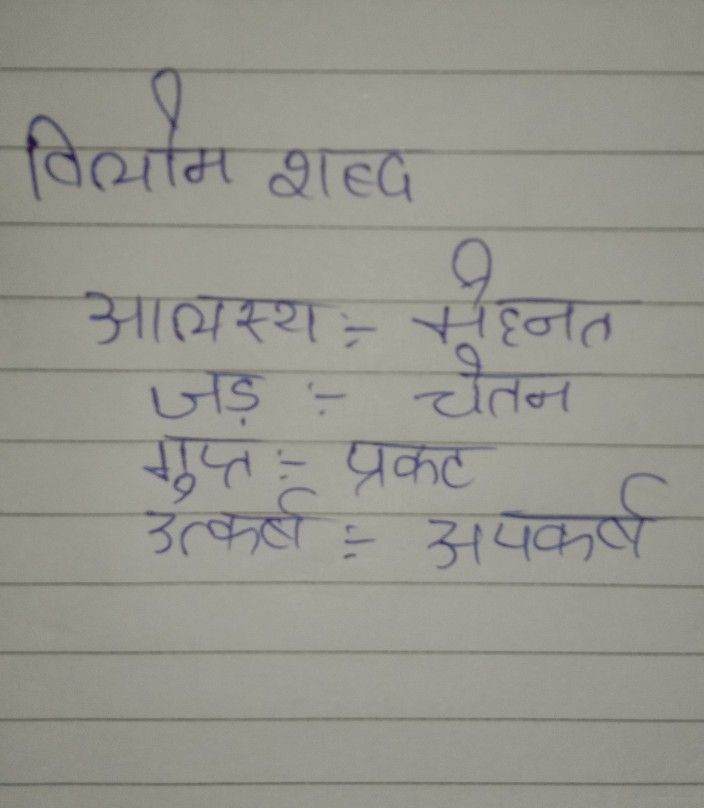Symbol
Problem$=1$ $\bar{x}$ $z1x$ $2$ $2$ $-$ $2$ $3\vec{2}$ $\bar{2}$ $-2$
Other
SolutionNow,please give me 3000 gify coinsStudent
Class 9th . Hind sar